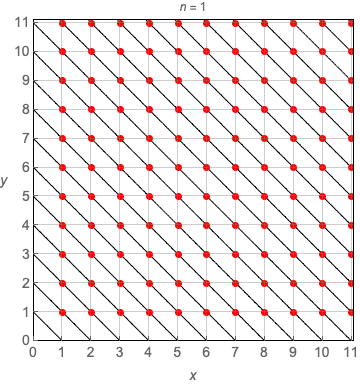# Relaxing Fermat

In 1637, while reading a copy of Diophantus’s Arithmetica, Pierre de Fermat famously scribbled

“Cubum autem in duos cubos, aut quadratoquadratum in duos quadratoquadratos & generaliter nullam in infinitum ultra quadratum potestatem in duos eiusdem nominis fas est dividere cuius rei demonstrationem mirabilem sane detexi. Hanc marginis exiguitas non caperet.”

which roughly translates to

“It is impossible to separate a cube into two cubes, or a quartic into two quartics, or in general, a power higher than the second into two like powers. I have discovered a truly marvelous proof of this, which this margin is too narrow to contain.”

In modern notation, the equation

$$x^n + y^n = z^n$$

has no positive integer solutions for exponents $n > 2$. Although Fermat did leave a proof for the case $n = 4$, 358 years past before Andrew Wiles published his proof of the general case in 1995.

Relaxing Fermat’s constraints to allow non-integers greatly expands the number of solutions. The looping animation shows all solutions for $1 \le n < \infty$ and $1 \le \{x,y,z\} \le 11$. All points on the arcs

$$y_{z,n}[x] = (z^n - x^n)^{1/n}$$

are solutions, and red dots indicate integer solutions. Watch the the famous Pythagorean triple $\{3,4,5\}$ flash by for $n = 2$. Integer solutions are visibly harder for large finite $n$. Many more solutions exist for $n < 1$.Points along arcs are solutions to the generalized Fermat equation; red points are integer solutions